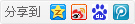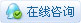##帐号 自动登录 找回密码 密码 立即注册→加入我们
 搜索 热搜: 下载 VB C 实现 编写

# 【VFB】变量和数据类型（VFB教程2-3）

51主题 642积分

UID
3260

7

12 点

502 个

1 次

0 份

22 小时

2017-12-26发表于 2018-2-26 21:13:37 | 显示全部楼层 |阅读模式

### 欢迎访问技术宅的结界，请注册或者登录吧。

x

[Visual Basic] 纯文本查看 复制代码
'--------------------------------------------------------------------------------
Function FORM1_COMMAND1_BN_CLICKED ( _
ControlIndex     As Long, _      ' 控件数组的索引
hWndForm         As HWnd, _      ' 窗体句柄
hWndControl      As HWnd, _      ' 控件句柄
idButtonControl  As Long   _     ' 按钮的标识符
) As Long
Dim FirstNumber As Integer
Dim Shared SecondNumber As Integer

FirstNumber = 1
SecondNumber = 2

PrintConstants ()
Print FirstNumber, SecondNumber, ThirdNumber '这将打印1 2 0
End Function
'--------------------------------------------------------------------------------
Sub PrintConstants ()
Dim ThirdNumber As Integer
ThirdNumber = 3
Print FirstNumber, SecondNumber, ThirdNumber '这将打印0 2 3
End Sub

[Visual Basic] 纯文本查看 复制代码
Const FirstNumber = 1
Const SecondNumber = 2
Const FirstString = "第一个字符串"
'--------------------------------------------------------------------------------
Function FORM1_COMMAND1_BN_CLICKED ( _
ControlIndex     As Long, _      ' 控件数组的索引
hWndForm         As HWnd, _      ' 窗体句柄
hWndControl      As HWnd, _      ' 控件句柄
idButtonControl  As Long   _     ' 按钮的标识符
) As Long
Print FirstNumber, SecondNumber '这将打印1 2
Print FirstString '这将打印第一个字符串。
PrintConstants ()
End Function
'--------------------------------------------------------------------------------
Sub PrintConstants ()
Print FirstNumber, SecondNumber '这也将打印1 2
Print FirstString '这也将打印第一个字符串。
End Sub

[Visual Basic] 纯文本查看 复制代码
' Create an array of 3 elements all having the value zero (0.0f).
Dim array(1 To 3) As Single

' Assign a value to the first element.
array(1) = 1.2

' Output the values of all the elements ("1.2 0 0").
For position As Integer = 1 To 3
Print array(position)
Next

[Visual Basic] 纯文本查看 复制代码
' Declares and initializes an array of four integer elements.
Dim array(3) As Integer = { 10, 20, 30, 40 }

' Outputs all of the element values (" 10 20 30 40").
For position As Integer = LBound(array) To UBound(array)
Print array(position) ;
Next

[Visual Basic] 纯文本查看 复制代码
' Creates a fixed-length array that holds 5 single elements.
Const totalSingles = 5
Dim flarray(1 To totalSingles) As Single

' Creates an empty variable-length array that holds integer values.
Dim vlarray() As Integer

' Resizes the array to 10 elements.
ReDim vlarray(1 To 10) As Integer

[Visual Basic] 纯文本查看 复制代码
' Take Care while initializing multi-dimensional array
Dim As Integer multidim(1 To 2,1 To 5) = {{0,0,0,0,0},{0,0,0,0,0}}

[Visual Basic] 纯文本查看 复制代码
'' Defines a one-dimensional fixed-length array of type INTEGER having automatic storage.
Dim arrayOfIntegers(69) As Integer

'' Defines a one-dimensional fixed-length array of type SHORT having static storage.
Static arrayOfShorts(420) As Short

[Visual Basic] 纯文本查看 复制代码
Dim a(1) As Integer  '' 1-dimensional, 2 elements (0 and 1)
Dim b(0 To 1) As Integer  '' 1-dimensional, 2 elements (0 and 1)
Dim c(5 To 10) As Integer  '' 1-dimensional, 5 elements (5, 6, 7, 8, 9 and 10)

Dim d(1 To 2, 1 To 2) As Integer  '' 2-dimensional, 4 elements: (1,1), (1,2), (2,1), (2,2)
Dim e(255, 255, 255, 255) As Integer '' 4-dimensional, 256 * 256 * 256 * 256 elements

[Visual Basic] 纯文本查看 复制代码
Const myLowerBound = -5
Const myUpperBound = 10

'' Declares a one-dimensional fixed-length array, holding myUpperBound - myLowerBound + 1 String objects.
Dim arrayOfStrings(myLowerBound To myUpperBound) As String

'' Declares a one-dimensional fixed-length array of bytes,
'' big enough to hold an INTEGER.
Dim arrayOfBytes(0 To SizeOf(Integer) - 1) As Byte

[Visual Basic] 纯文本查看 复制代码
'' Declares a one-dimensional variable-length array of integers, with initially 2 elements (0 and 1)
ReDim a(0 To 1) As Integer

'' Declares a 1-dimensional variable-length array without initial bounds.
'' It must be resized using Redim before it can be used for the first time.
Dim b(Any) As Integer

'' Same, but 2-dimensional
Dim c(Any, Any) As Integer

Dim myLowerBound As Integer = -5
Dim myUpperBound As Integer = 10

'' Declares a 1-dimensional variable-length array by specifying variable (non-constant) boundaries.
'' The array will have myUpperBound - myLowerBound + 1 elements.
Dim d(myLowerBound To myUpperBound) As Integer

'' Declares a variable-length array whose amount of dimensions will be determined
'' by the first Redim or array access found. The array has no initial bounds and must
'' be resized using Redim before it can be used for the first time.
Dim e() As Integer

[Visual Basic] 纯文本查看 复制代码
'' Define an empty 1-dimensional variable-length array of SINGLE elements...
Dim array(Any) As Single

'' Resize the array to hold 10 SINGLE elements...
ReDim array(0 To 9) As Single

'' The data type may be omitted when resizing:
ReDim array(10 To 19)

[Visual Basic] 纯文本查看 复制代码
Index        Data
1              5
2              2
3              6
4              5
5              9
6              1
7              0
8              4
9              5
10             7

[Visual Basic] 纯文本查看 复制代码
Print myArray(5)

9

[Visual Basic] 纯文本查看 复制代码
myArray(3) = 0

[Visual Basic] 纯文本查看 复制代码
Print myArray(3)

0

[Visual Basic] 纯文本查看 复制代码
    Dim a As Integer
For a = 1 To 10
myArray(a) = 0
Next a

[Visual Basic] 纯文本查看 复制代码
    Dim Index As Integer
Dim Value As Integer
index = Int(Rnd(1) * 10) + 1 'This line will simply return a random value between 1 and 10
Value = Int(Rnd(1) * 10) + 1 'This line will do the same
myArray(index) = Value

[Visual Basic] 纯文本查看 复制代码
Dim Numbers(1 To 10) As Integer
Dim Shared OtherNumbers(1 To 10) As Integer
Dim a As Integer

Numbers(1) = 1
Numbers(2) = 2
OtherNumbers(1) = 3
OtherNumbers(2) = 4

PrintArray ()

For a = 1 To 10
Print Numbers(a)
Next a

Print OtherNumbers(1)
Print OtherNumbers(2)
Print OtherNumbers(3)
Print OtherNumbers(4)
Print OtherNumbers(5)
Print OtherNumbers(6)
Print OtherNumbers(7)
Print OtherNumbers(8)
Print OtherNumbers(9)
Print OtherNumbers(10)

Sub PrintArray ()
Dim a As Integer
For a = 1 To 10
Print otherNumbers(a)
Next a
End Sub

Byte和UByte

Short和UShort

Long和Ulong

Integer和UInteger

LongInt和ULongInt

Single

Double

Const

Pointer和Ptr

Unsigned

String

ZString

WString

Object

 类型 大小在位 格式 最小值 最大值 字面后缀 SIG。数字 BYTE 8 有符号整数 -128 +127 2+ UBYTE 8 无符号整数 0 +255 2+ SHORT 16 有符号整数 -32768 +32767 4+ USHORT 16 无符号整数 0 65535 4+ LONG 32 有符号整数 -2147483648 +2147483647 &, l 9+ ULONG 32 无符号整数 0 4294967295 ul 9+ INTEGER 32/64 ［*］ 有符号整数 ［*］ ［*］ % ［*］ UINTEGER 32/64 ［*］ 无符号整数 ［*］ ［*］ u ［*］ LONGINT 64 有符号整数 -9 223 372 036 854 775 808 +9 223 372 036 854 775 807 ll 18+ ULONGINT 64 无符号整数 0 +18 446 744 073 709 551 615 ULL 19+ SINGLE 32 浮点 ［**］+/-1.401 298 E-45 ［**］+/-3.402 823 E+38 !, f 6+ DOUBLE 64 浮点 ［**］+/-4.940 656 458 412 465 E-324 ［**］+/-1.797 693 134 862 316 E+308 #, d 15+

［*］ 整数和UInteger数据类型随平台而异，与指针的大小相匹配。
［**］ 浮点类型Single和Double的最小值和最大值分别为最接近零的值，最接近正负无穷大的值。

 类型 字符大小（字节） 最小尺寸（以字符） 最大尺寸（以字符） 字面后缀 string 1 0 ［**］+2147483647 \$ zstring 1 0 ［**］+2147483647 [N/A] WSTRING ［*］ ［*］0 [*,**]+2147483647 [N/A]

［*］ Unicode或“wide”，字符大小和可用性随平台而异。
［**］ 所有运行时库字符串过程都会获取大小和位置的整数值。 实际的最大尺寸将随存储位置和/或平台而变化（较小）。

 最大下标范围 每个维度的最大元素 最小/最大尺寸 最大大小（以字节为单位） ［*］[-2147483648, +2147483647] ［*］+2147483647 1/9 ［*］+2147483647

［*］ 所有运行时库数组过程都会为下标和索引生成整数值。 实际限制将随维度数量，元素大小，存储位置和/或平台而变化（较小）。本版积分规则 回帖并转播 回帖后跳转到最后一页|申请友链||Archiver|手机版|小黑屋|技术宅的结界 ( 滇ICP备16008837号 )|网站地图

GMT+8, 2020-12-3 05:42 , Processed in 0.095023 second(s), 32 queries , Gzip On.